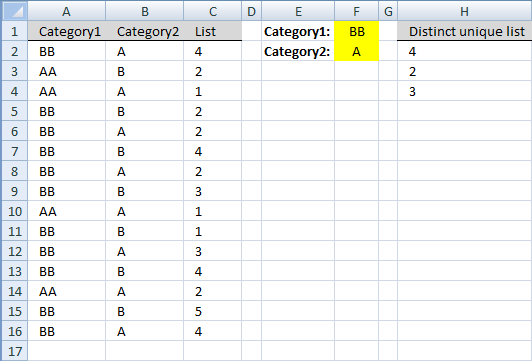Author: Oscar Cronquist Article last updated on November 20, 2018

Question: How do I create a unique distinct list where other columns meet two criteria using excel array formula?### Excel 2007 array formula in H2:

=IFERROR(INDEX(List, MATCH(0, COUNTIF(H1:\$H\$1, List)+IF(Category2<>Criteria2, 1, 0)+IF(Category1<>Criteria1, 1, 0), 0)), "")

### How to create an array formula

1. Copy (Ctrl + c) and paste (Ctrl + v) array formula into formula bar.
2. Press and hold Ctrl + Shift.
3. Press Enter once.
4. Release all keys.

Copy cell H2. Paste down to cell H16.

### Earlier Excel versions, array formula in H2:

=IF(ISERROR(INDEX(List, MATCH(0, COUNTIF(J1:\$J\$1, List)+IF(Category2<>Criteria2, 1, 0)+IF(Category1<>Criteria1, 1, 0), 0))),"",INDEX(List, MATCH(0, COUNTIF(J1:\$J\$1, List)+IF(Category2<>Criteria2, 1, 0)+IF(Category1<>Criteria1, 1, 0), 0)))

Copy cell H2. Paste down to cell H16.

### Named ranges

List (C2:C16)
Category1 (A2:A16)
Category2 (B2:B16)
Criteria1 (F1)
Criteria2 (F2)

What is named ranges?

### How to create named ranges

1. Select cell range C2:C16
2. Type List in name box

### Explaining excel 2007 array formula in cell H2

Step 1 - Create boolean array with values indicating unique distinct values

=IFERROR(INDEX(List, MATCH(0, COUNTIF(H1:\$H\$1, List)+IF(Category2<>Criteria2, 1, 0)+IF(Category1<>Criteria1, 1, 0), 0)), "")

COUNTIF(H1:\$H\$1, List)

becomes

COUNTIF("Distinct unique list", {4; 2; 1; 2; 2; 4; 2; 3; 1; 1; 3; 4; 2; 5; 7})

and returns {0; 0; 0; 0; 0; 0; 0; 0; 0; 0; 0; 0; 0; 0; 0}.

How to use the COUNTIF function

Counts the number of cells that meet a specific condition.

Step 2 - Create boolean array with values indicating first criterion

=IFERROR(INDEX(List, MATCH(0, COUNTIF(H1:\$H\$1, List)+IF(Category2<>Criteria2, 1, 0)+IF(Category1<>Criteria1, 1, 0), 0)), "")

IF(Category1<>Criteria1, 1, 0)

becomes

IF({"BB"; "AA"; "AA"; "BB"; "BB"; "BB"; "BB"; "BB"; "AA"; "BB"; "BB"; "BB"; "AA"; "BB"; "BB"}<>"BB", 1, 0)

and returns {0;1;1;0;0;0;0;0;1;0;0;0;1;0;0}.

How to use the IF function

Checks if a logical expression is met. Returns a specific value if TRUE and another specific value if FALSE.

Step 3 - Create boolean array with values indicating second criterion

=IFERROR(INDEX(List, MATCH(0, COUNTIF(H1:\$H\$1, List)+IF(Category2<>Criteria2, 1, 0)+IF(Category1<>Criteria1, 1, 0), 0)), "")

IF(Category2<>Criteria2, 1, 0)

becomes

IF({"A"; "B"; "A"; "B"; "A"; "B"; "A"; "B"; "A"; "B"; "A"; "B"; "A"; "B"; "C"}<>"A", 1, 0)

and returns {0; 1; 0; 1; 0; 1; 0; 1; 0; 1; 0; 1; 0; 1; 1}.

Step 4 - Add boolean arrays

=IFERROR(INDEX(List, MATCH(0, COUNTIF(H1:\$H\$1, List)+IF(Category2<>Criteria2, 1, 0)+IF(Category1<>Criteria1, 1, 0), 0)), "")

becomes

=IFERROR(INDEX(List, MATCH(0,{0; 0; 0; 0; 0; 0; 0; 0; 0; 0; 0; 0; 0; 0; 0}+{0; 1; 0; 1; 0; 1; 0; 1; 0; 1; 0; 1; 0; 1; 1}+{0;1;1;0;0;0;0;0;1;0;0;0;1;0;0}, 0)), "")

becomes

=IFERROR(INDEX(List, MATCH(0,{0;2;1;1;0;1;0;1;1;1;0;1;1;1;1}, 0)), "")

Step 4 - Return relative position of the first zero (0) in array

=IFERROR(INDEX(List, MATCH(0,{0;2;1;1;0;1;0;1;1;1;0;1;1;1;1}, 0)), "")

becomes

=IFERROR(INDEX(List, 1), "")

How to use the MATCH function

Identify the position of a value in an array.

Step 5 - Return a value or reference of the cell at the intersection of a particular row and column

=IFERROR(INDEX(List, 1), "")

becomes

=IFERROR(4, "")

How to use the INDEX function

Gets a value in a specific cell range based on a row and column number.

Step 6 - Check if expression returns an error

=IFERROR(4, "")

returns number 4 in cell H2.

How to use the IFERROR function

If the value argument returns an error, the value_if_error argument is used. If the value argument does NOT return an error, the IFERROR function […]